# Curves and Ruled Surfaces over Curves

Curves are parametrized by the time in [0,1].

## Polyline and Bezier Curves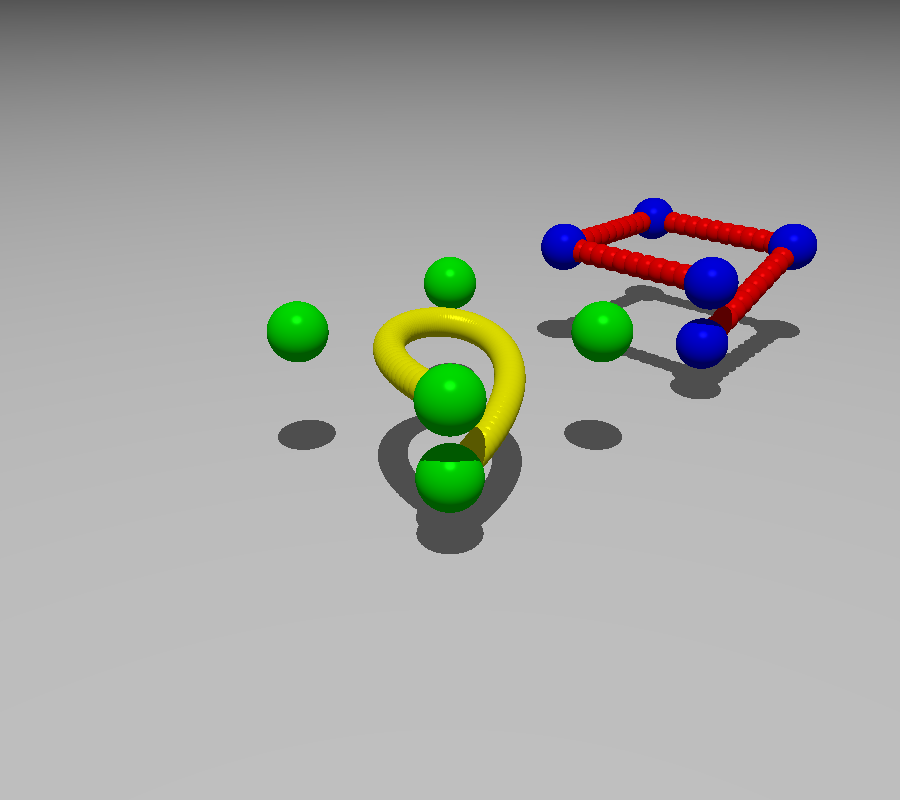The following sequence builds the basic usable curves, namely a Bezier curve and a Polyline. Since a curve is infinitly thin, it is not possible to see it even if camera.filmActors=True. We use the method show() to add spheres along the curve to visualize it. The options of show() allow to choose a different color for the control points and for the sphere along the curve.

  ground=plane(Z,origin-Z)
ground.color="Gray"
curve1=BezierCurve([origin,-X+Y,X+Y,X-Y,-X-Y-.5*Z])
curve1.show() # use default arguments
curve2=Polyline([origin,-X+Y,X+Y,X-Y,-X-Y-.5*Z])
# For the polyline, we don't use the default options
curve2.show(radius=0.1,steps=50,color="Red",color2="Blue")
curve2.translate(2*X+2*Y)
camera=Camera()
camera.file="polylineAndBezier.pov"
camera.actors=[ground,curve1,curve2]
camera.zoom(.32)
camera.shoot
camera.show

Constructor in absolute or relative notations


The constructor BezierCurve([c0,c1,c2...]) defines the control points. If the element ci is a point, it is equal to , the control point p(i) is defined by p(i)=ci. If ci is a vector, then p(i) is defined as p(i)=p(i-1)+ci, ie. ci is the relative position with respect to the preceding point.

Similarly, the control points of a polyline may be defined in absolute terms by a point, or in relative terms with respect to the preceding point using a vector.

## Interpolation and Piecewise curves

Usually we want an smooth interpolation curve. Thus neither the Bezier curve ( it does not pass through the control points) neither the polyline (not smooth ) are suitable.

The following sequence builds a smooth curve C through the same points as above.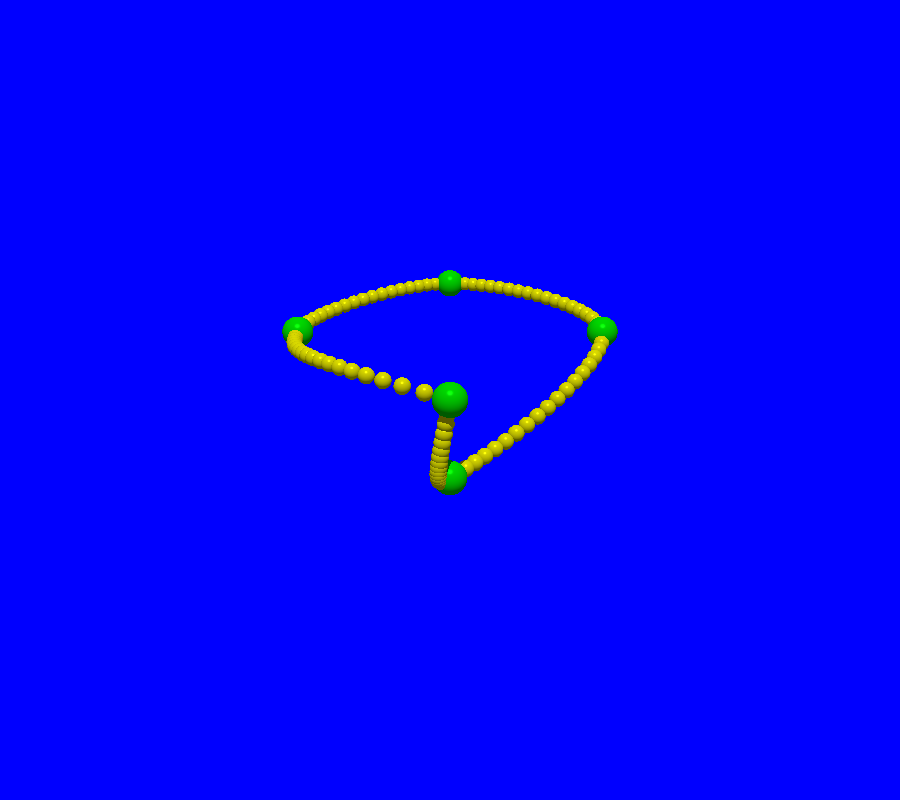relativeList=([origin,-X+Y,X+Y,X-Y,-X-Y-.5*Z])
controlPoints=ParametrizedCurve.relativeToAbsolute(relativeList)
curve=PiecewiseCurve.fromInterpolation(controlPoints).show()
# The spheres for the 4 points prescribed
greenSpheresOnControlPoints=[ Sphere(cp,.2).colored("Green") for cp in controlPoints]

camera=Camera()
camera.file="curve.pov"
camera.filmAllActors=True
camera.zoom(0.32)
camera.shoot
camera.show


## Closed curves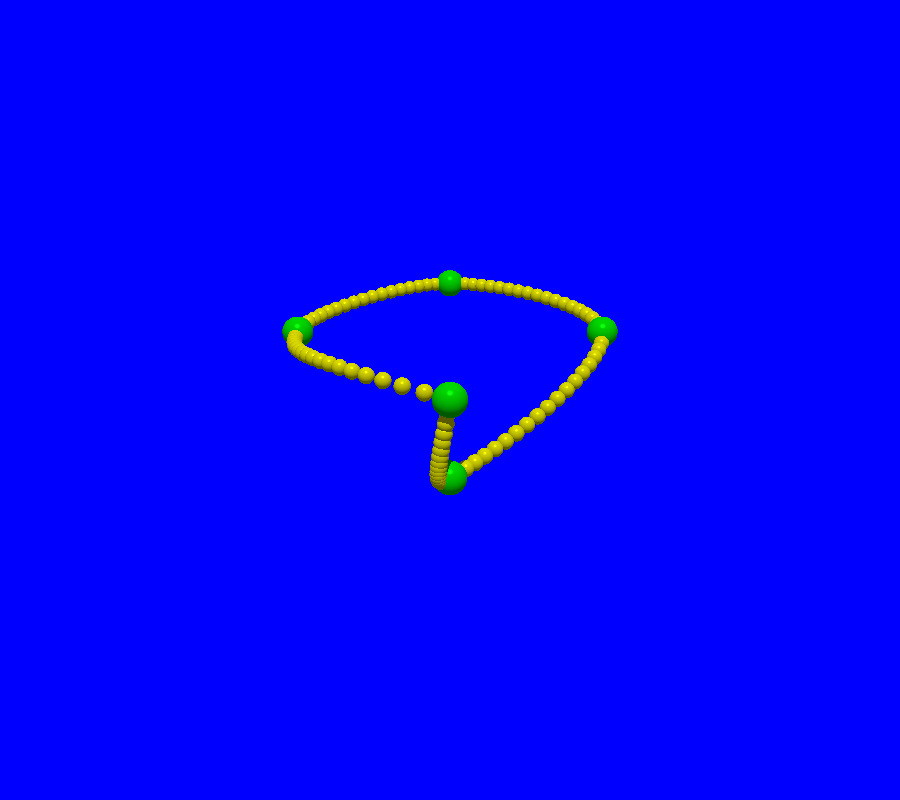Now, we add from our last curve a new control point to “close the curve”. The curve admits “an angle” at the locus where the end of the curve joins the starting point.

relativeList=([origin,-X+Y,X+Y,X-Y,-X-Y-.5*Z,origin])
controlPoints=ParametrizedCurve.relativeToAbsolute(relativeList)
curve=PiecewiseCurve.fromInterpolation(controlPoints).show(radius=.05)
# The spheres for the 4 points prescribed
greenSpheresOnControlPoints=[ Sphere(cp,.1).colored("Green") for cp in controlPoints]

camera=Camera()
camera.file="curveClosed.pov"
camera.filmAllActors=True
camera.zoom(0.32)
camera.shoot
camera.show


As we see, this is not smoot junction at the locus where the ending point joins the starting point. Thus we add the argument “closedCurve=True”. This allows the curve to be “smooth”, ie. differentiable in technical terms.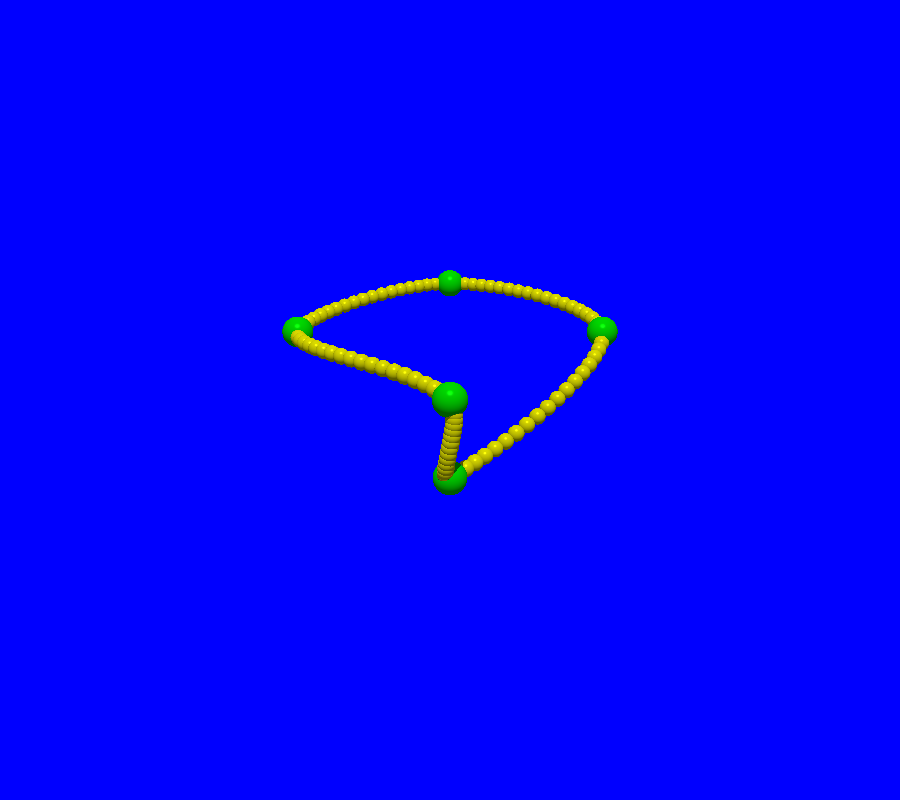relativeList=([origin,-X+Y,X+Y,X-Y,-X-Y-.5*Z,origin])
controlPoints=ParametrizedCurve.relativeToAbsolute(relativeList)
curve=PiecewiseCurve.fromInterpolation(controlPoints,closedCurve=True).show(radius=.05)
# The spheres for the 4 points prescribed
greenSpheresOnControlPoints=[ Sphere(cp,.1).colored("Green") for cp in controlPoints]

camera=Camera()
camera.file="curveClosedSmooth.pov"
camera.filmAllActors=True
camera.zoom(0.32)
camera.shoot
camera.show


## Ruled Surfaces built on curves

It is possible to fill a closed curve to get a surface. For instance, if we fill a circle, we get a disk. The calling sequence is RuledSurface.fromCurveFilling(curve), as illustrated in the red example below.

It is possible to construct a ruled surface by joining the points C(t) and D(t) of two curves C and D. The following example illustrates that the result depends on the parametrization of C and D . The violet join uses the same curves as the green join, but with a different parametrization equivalent to a rotation of the bottom curve. It looks like we have applied a torsion to an aluminium can. The violet join also illustrates that we can optionally add caps at the extremities of the join.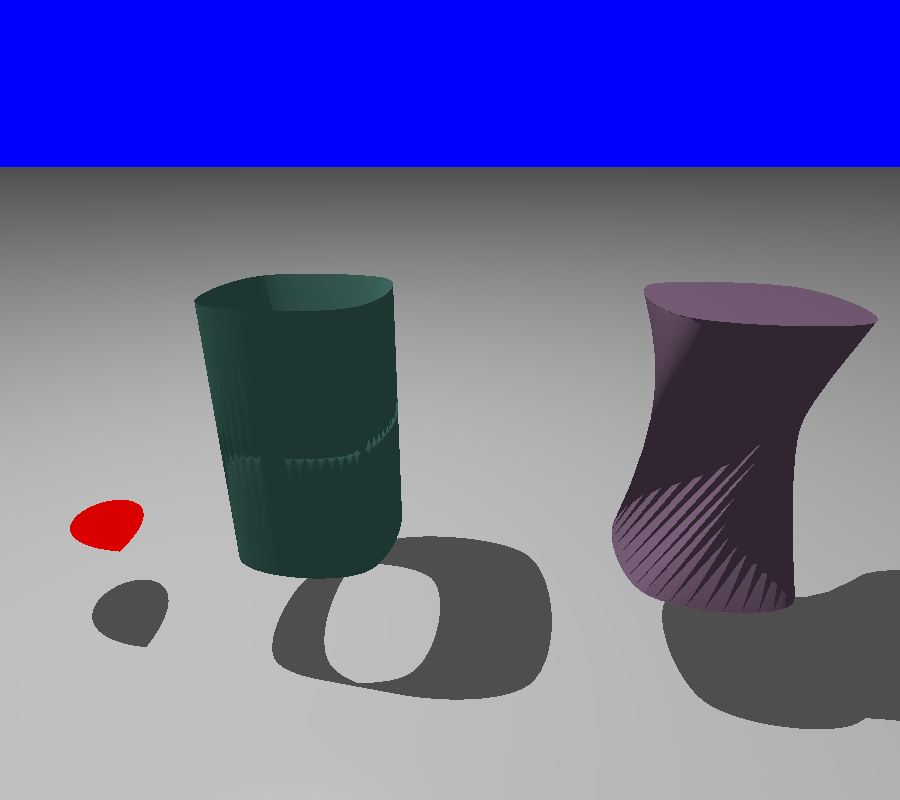ground=plane(Z,origin-Z)
ground.color="Gray"

#Filling a Bezier curve in Red
curve0=BezierCurve([origin,-X,+Y,+X,origin])
curveFilling=RuledSurface.fromCurveFilling(curve0,quality=2)
curveFilling.color="Red"
curveFilling.translate(-2*X)

# The green join
curve1=PiecewiseCurve.fromInterpolation([origin,-X,+Y,+X,origin], closedCurve=True)
curve1.show(radius=0.1,steps=10,color="SpicyPink",color2="Yellow")
curve2=curve1.copy().translate(2*Z)
tube=RuledSurface(curve1,curve2,quality=8)
tube.color="HuntersGreen"

#The violet join
curve3=curve1.copy().translate(3*X)
curve4=curve2.copy().translate(3*X)
def g(t):
return (t+0.25) %1
curve3.reparametrize(g)
tubeWithRotatedBottom=RuledSurface.fromJoinAndCaps(curve3,curve4,quality=5)
tubeWithRotatedBottom.color="Violet"

camera=Camera()
camera.filmAllActors=False
camera.file="ruledSurface.pov"
camera.location=origin-3.3*Y+2.8*Z+X
camera.zoom(.22)
camera.povraylights="light_source {<-2,0,6.8> color White " + "}\n\n"
camera.lookAt=.5*(curve1(0.5)+curve4(0.5))
camera.actors=[curveFilling,tube,tubeWithRotatedBottom,ground]
camera.shoot
camera.show